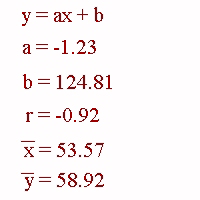### CFA Practice Question

There are 208 practice questions for this study session.

### CFA Practice Question

If you run linear regression on a set x-y data and obtain the results shown below then the predicted y-value for x = 60 is ______.A. 58.92
B. 51.01
C. 124.81